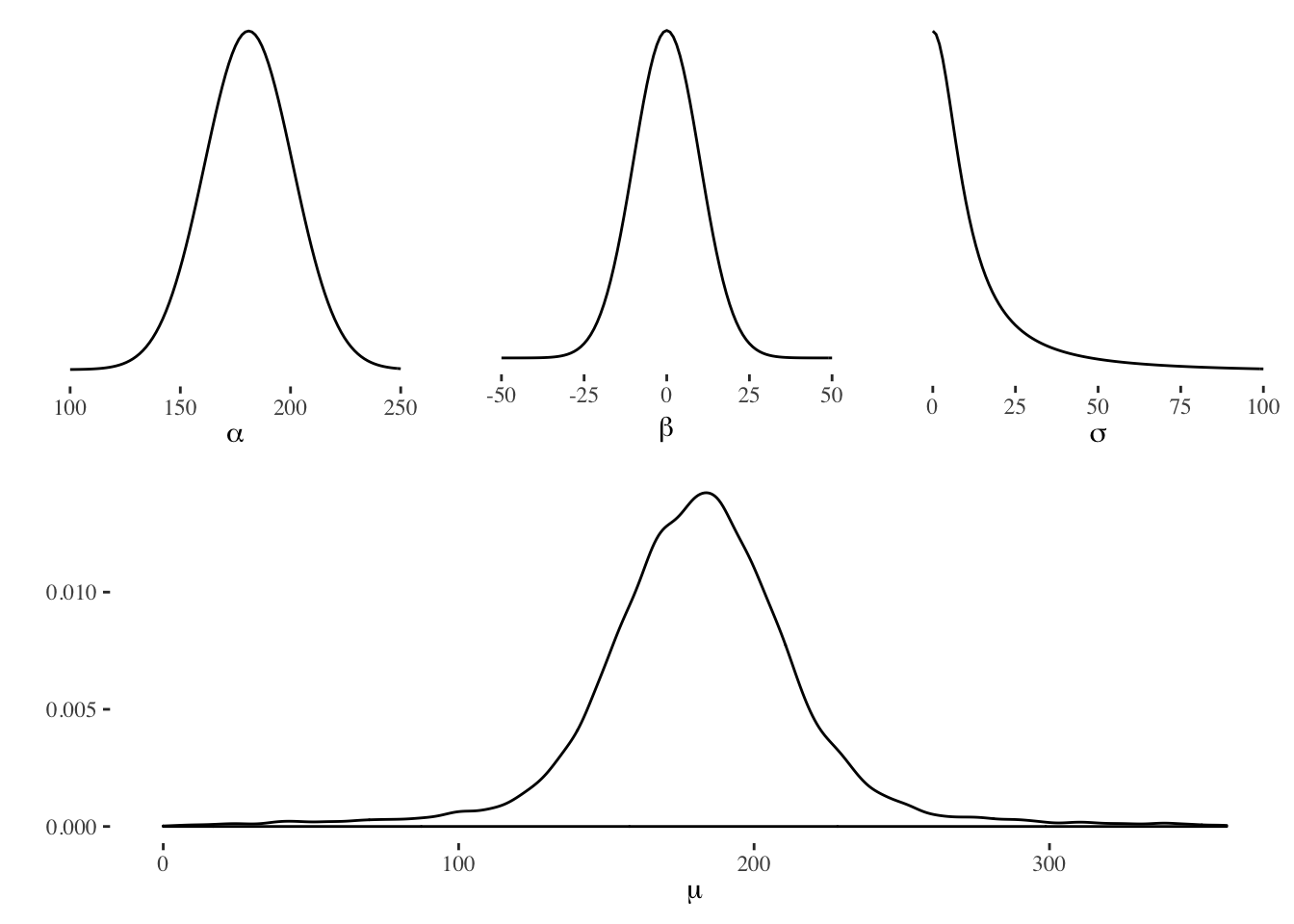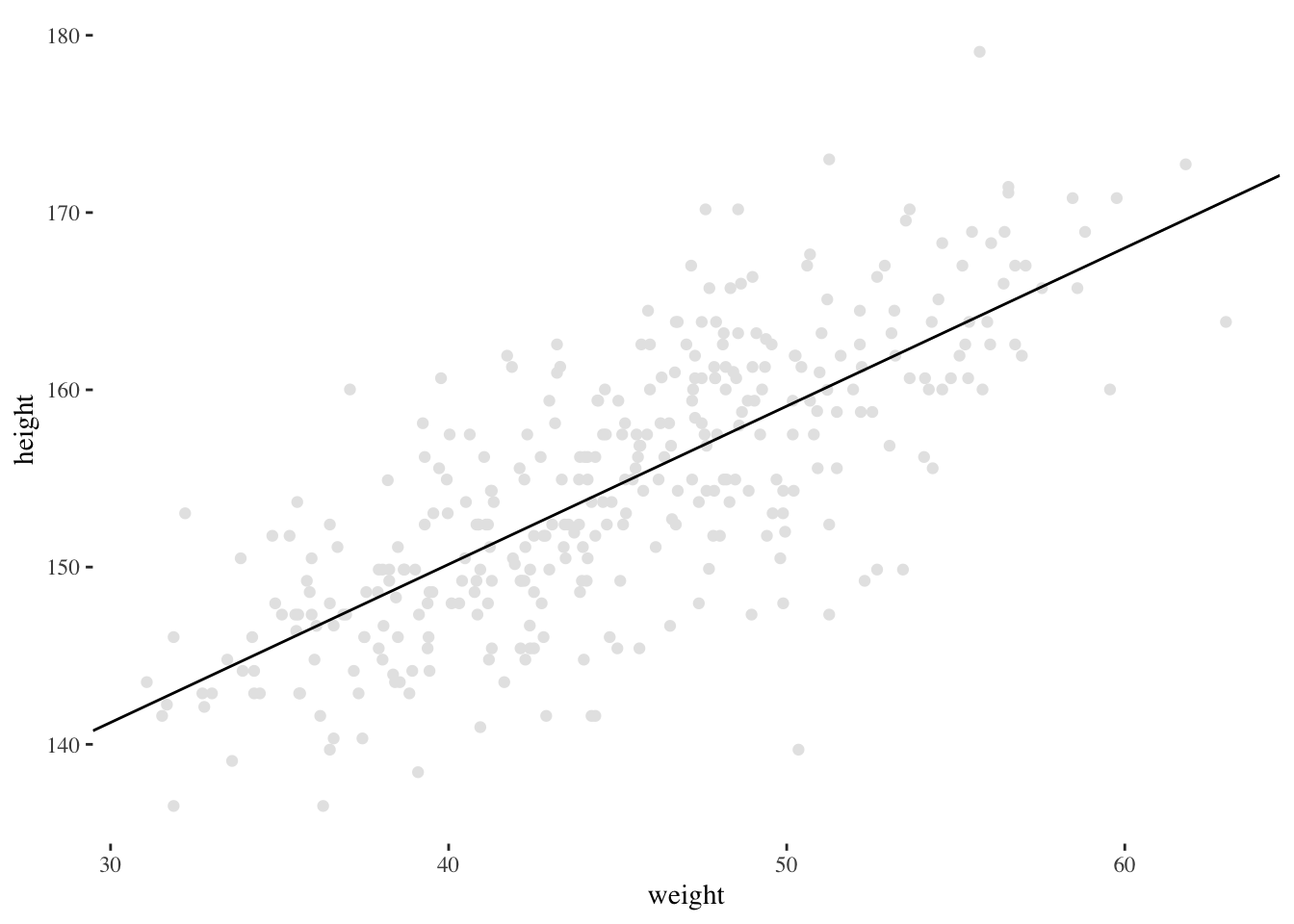## Prior predictive analysis

We will make use of a number of R packages. Both rethinking and brms allows us to develop models which we then run using Stan. In this example we’ll see both being used. Make sure that you have the following installed (including http://www.mc-stan.org):

library(rethinking)
library(brms)
library(tidyverse)
library(ggthemes)
library(ggpubr)

Let’s start by attaching the data which we will use for our example. This data comes from the rethinking package and according to (McElreath 2016) consists of:

partial census data for the Dobe area !Kung San, compiled from interviews conducted by Nancy Howell in the late 1960s.

data(Howell1)
d <- Howell1

Let us keep only subjects that are >= 18 years of age.

d2 <- d[ d\$age >= 18 , ]

Sample randomly for our three priors on $$\alpha$$, $$\beta$$, and $$\sigma$$, and plot the results. Additionally, let us plot the combination of all priors to represent the combined effect of the priors on $$\mu$$.So, above we see each prior and the combination of the priors for $$\mu$$. In short, we assume $$alpha \sim \mathcal{N}(181,20)$$, $$\beta \sim \mathcal{N}(0,10)$$, and $$\sigma \sim \text{Half-Cauchy}(0,10)$$.

We can now check for sanity issues concerning our priors. For example, how many people are <53 or >272 cm? Well, it’s easy to do now when we have a “posterior”, i.e., the answer is 1.57% and 1.58%, respectively.

In short, we still allow extreme values, but we don’t allow too many absurd values. To summarize, our model now looks like, $\begin{eqnarray} \text{height}_i & \sim & \mathcal{N}(\mu_i,\sigma)\\ \mu_i & \sim & \alpha + \beta_w \times \text{weight}_i\\ \alpha & \sim & \mathcal{N}(181, 20)\\ \beta_w & \sim & \mathcal{N}(0, 10)\\ \sigma & \sim & \text{Half-Cauchy}(0, 10) \end{eqnarray}$ Let us now go through this line by line. First, we claim that height has a Normal distribution with mean $$\mu$$ and standard deviation $$\sigma$$. The subset $$i$$ in height, weight and $$\mu$$ is an indication that this holds for each height we have in the data set. But why Normal? Well, there are ontological and epistemological reasons for this, but in short: if we add together random values from the same distribution it converges to a normal distribution.

The next line shows our linear model. We have an intercept $$\alpha$$, together with the slope $$\beta_w$$. We want to estimate these two parameters using the data: height and weight. Height is the outcome and weight is the predictor.

Next, we have our priors. The $$\alpha$$ parameter is the intercept, and hence our expected mean. What we are saying is that we have prior knowledge, i.e., we believe that the mean will gravitate towards 181 cm. Why 181? Well, this is the average height of the three authors of this exercise. In addition, we say that we can expect the mean to vary with a standard deviation of 20. For $$\beta_w$$ our prior indicates that the slope has a mean of 0 and a standard deviation of 10. We could also set a different prior here, e.g., we have a feeling that an increase in weight also leads to an increase in height, but for now we will leave it at that. Finally, we have a prior on $$\sigma$$ known as Half-Cauchy. The Half-Cauchy is a common prior for $$\sigma$$ and is roughly a Normal distribution cut in half, i.e., we do not allow negative values on $$\sigma$$. In the end, if we have enough evidence (data) it will swamp the priors. (Feel free to mess around in the code and see what happens when you change the priors.)

## Model and execution (rethinking)

The below model is declared using the “rethinking” package and as you see it closely follows our model declaration we did in math above. In the examples we show here we have not standardized our predictor values. This is something one, generally speaking, always should do, mainly to help ‘Stan’ sample values.

model <- ulam(
alist(
height ~ normal(mu, sigma),
mu <- alpha + beta_w * weight,
alpha ~ normal(181, 20),
beta_w ~ normal(0,10),
sigma ~ cauchy(0,10)
), data = d2, chains = 4, cores = 4, iter = 1e4, control=list(max_treedepth=13)
)

After having run the model we can now look at the posterior. Let’s use precis() from the rethinking package, which summarizes the posterior for us.

precis(model)
##               mean         sd        5.5%       94.5%    n_eff      Rhat
## alpha  114.4591868 1.90351257 111.4579732 117.4935463 5778.879 1.0005265
## beta_w   0.8924486 0.04187359   0.8257596   0.9586349 5780.948 1.0005421
## sigma    5.1012668 0.19397810   4.8049909   5.4237178 8953.301 0.9999984

What we see is that the intercept ($$\alpha$$) is approximately 114.46 cm (the !Kung people seems to be short), and there’s a positive $$\beta_w$$ parameter, i.e., weight and height goes partly hand in hand. In this particular case we’re saying that a person 1 kg heavier is expected to be 0.89 cm taller

Let’s now plot our data and the linear prediction we calculated.## Model and execution (brms)

The book McElreath (2016) wrote is a good introduction to Bayesian data analysis. However, more straightforward tools exist, e.g., brms which uses the common lme4 syntax for declaring models. Below we declare and run the same model in brms

model_brms <- brm(bf(height ~ 1 + weight),
prior = c(prior(normal(181,20), class = "Intercept"),
prior(normal(0,10), class="b"),
prior(cauchy(0,10), class="sigma")),
data=d2, chains=4, cores=4, iter=1e4)
## Compiling the C++ model
## Start sampling

With brms we can use the summary() function to view a summary of the posterior probability distribution. As you see below, these values are very much the same as when we used rethinking.

summary(model_brms)
##  Family: gaussian
##   Links: mu = identity; sigma = identity
## Formula: height ~ 1 + weight
##    Data: d2 (Number of observations: 352)
## Samples: 4 chains, each with iter = 10000; warmup = 5000; thin = 1;
##          total post-warmup samples = 20000
##
## Population-Level Effects:
##           Estimate Est.Error l-95% CI u-95% CI Eff.Sample Rhat
## Intercept   113.88      1.93   110.07   117.64      18411 1.00
## weight        0.91      0.04     0.82     0.99      18438 1.00
##
## Family Specific Parameters:
##       Estimate Est.Error l-95% CI u-95% CI Eff.Sample Rhat
## sigma     5.10      0.20     4.74     5.51      18158 1.00
##
## Samples were drawn using sampling(NUTS). For each parameter, Eff.Sample
## is a crude measure of effective sample size, and Rhat is the potential
## scale reduction factor on split chains (at convergence, Rhat = 1).

Let’s now plot the empirical data, but this time we also plot the uncertainty by using $$\sigma$$. The dark line is the maximum a posteriori estimate of $$\mu$$. The two dark intervals show two different 95% uncertainty intervals. The dark gray interval shows the uncertainty around $$\mu$$. The light gray interval shows the uncertainty concerning all values, i.e., 95% of all values should be within this interval.